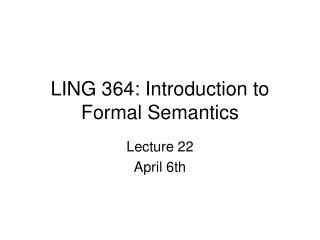# LING 364: Introduction to Formal Semantics - PowerPoint PPT PresentationDownload PresentationLING 364: Introduction to Formal Semantics

Download Presentation## LING 364: Introduction to Formal Semantics

- - - - - - - - - - - - - - - - - - - - - - - - - - - E N D - - - - - - - - - - - - - - - - - - - - - - - - - - -
##### Presentation Transcript

1. LING 364: Introduction to Formal Semantics Lecture 22 April 6th

2. Administrivia • Homework 5 • on quantification • out today • due next Thursday

3. Exercise 1 • Truth Tables and Prolog

4. Exercise 1 • Truth tables in Prolog • Example: • % implies(P,Q,Result) • implies(true,false,false). • implies(false,true,true). • implies(false,false,true). • implies(true,true,true). • % or(P,Q,Result) • or(true,true,true). • or(true,false,true). • or(false,true,true). • or(false,false,false). remember: Prolog variables are implicitly existentially quantified Show using a Prolog query that implies/3 and or/3 are not equivalent ?- implies(P,Q,R1), or(P,Q,R2), \+ R1 = R2. What should the outcome of this query be?

5. Exercise 1 • Homework Question A (3pts) • Using the Prolog query shown on the previous slide, • for what values of P and Q are implies/3 and or/3 incompatible? • Submit your run

6. Exercise 1 • Define truth table negation as follows: • % neg(P,\+ P). • neg(true,false). • neg(false,true). • Show using a Prolog query that P⇒Q is equivalent to ¬PvQ ?- implies(P,Q,R1), neg(P,NotP), or(NotP,Q,R2), \+ R1 = R2. What should the outcome of this query be?

7. Exercise 1 • Homework Question B (2pts) • Define truth table and/3 in Prolog • Homework Question C (4pts) • Using an appropriate Prolog query, and and/3, • Show that ¬(P∨Q) = ¬P∧¬Q (De Morgan’s Rule) • Submit your run

8. Exercise 1 • Homework Question D (4pts) • Using an appropriate Prolog query, • Show that ¬(P ∧Q) = ¬P∨¬Q • (another side of De Morgan’s Rule) • Submit your run

9. Exercise 1 • Summary • Submit answers to questions A through D • Points: • A: 3pts • B: 2pts • C: 4pts • D: 4pts • Total: 13 pts

10. Exercise 2 • Universal Quantification and Sets

11. Assume meaning grammar: s(M) --> qnp(M), vp(P), {predicate2(M,P)}. qnp(M) --> q(M), n(P), {predicate1(M,P)}. q((findall(_X,_P1,L1),findall(_Y,_P2,L2),subset(L1,L2))) --> [every]. n(woman(_)) --> [woman]. vp(M) --> v(M), np(X), {saturate2(M,X)}. v(likes(_X,_Y)) --> [likes]. np(ice_cream) --> [ice,cream]. saturate1(P,X) :- arg(1,P,X). saturate2(P,X) :- arg(2,P,X). subset([],_). subset([X|L1],L2) :- member(X,L2), subset(L1,L2). member(X,[X|_]). member(X,[_|L]) :- member(X,L). predicate1((findall(X,P,_),_),P) :- saturate1(P,X). predicate2((_,(findall(X,P,_),_)),P) :- saturate1(P,X). Exercise 2 every has semantics: {X: P1(X)} ⊆ {Y: P2(Y)} e.g. every woman likes ice cream {X: woman(X)} ⊆ {Y:likes(Y,ice_cream)}

12. Exercise 2 • Using the meaning grammar, we can compute a meaning expression for: • every woman likes ice cream using the Prolog query: • ?- s(M,[every,woman,likes,ice,cream],[]). • M = findall(A,woman(A),B),findall(C,likes(C,ice_cream),D),subset(B,D)

13. Exercise 2 • We can evaluate this meaning expression for various possible worlds using call/1 • For example, given the database: • woman(mary). woman(jill). • likes(john,ice_cream). likes(mary,ice_cream). • likes(jill,ice_cream). • we can evaluate: • ?- s(M,[every,woman,likes,ice,cream],[]), call(M). • the call is: • findall(A,woman(A),B),findall(C,likes(C,ice_cream),D),subset(B,D). • with • B and D being [mary,jill] and [john,mary,jill] respectively

14. Exercise 2 • Homework Question A (4pts) • Modify the meaning grammar to handle the sentence • John likes ice cream • Homework Question B (2pts) • EvaluateJohn likes ice cream against the database from the previous slide • Submit your run

15. Exercise 2 • Homework Question C (10pts) • Treating names as Generalized Quantifiers (see below), • Further modify the meaning grammar to handle the sentences • Every woman and John likes ice cream • John and every woman likes ice cream • Evaluate the sentences and submit your runs Define set union as follows: % L1 ∪ L2 = L3 “L3 is the union of L1 and L2” union(L1,L2,L3) :- append(L1,L2,L3). • Recall Lecture 21 • Example • every baby and John likes ice cream • [NP[NP every baby] and [NP John]] likes ice cream • ({X: baby(X)} ∪{X: john(X)}) ⊆ {Y: likes(Y,ice_cream)} • note: set union (∪) is the translation of “and”

16. Exercise 2 • Summary • Answer questions A, B and C • A: 4pts • B: 2pts • C: 10pts • Total: 16pts

17. Exercise 3 • Other quantifiers as generalized quantifiers

18. Exercise 3 • Other quantifiers can also be expressed using set relations between two predicates: Example: no: {X: P1(X)} ∩ {Y: P2(Y)} = ∅ ∩ = set intersection ∅ = empty set no man smokes {X: man(X)} ∩ {Y: smokes(Y)} = ∅ should evaluate to true for all possible worlds where there is no overlap between men and smokers men smokers

19. Exercise 3 • Other quantifiers can also be expressed using set relations between two predicates: Example: some: {X: P1(X)} ∩ {Y: P2(Y)} ≠∅ ∩ = set intersection ∅ = empty set some men smoke {X: man(X)} ∩ {Y: smokes(Y)} ≠ ∅ men smokers

20. Exercise 3 • Homework Question A (8pts) • Modify the meaning grammar given in exercise 2 to handle the sentence: • No woman likes ice cream • Evaluate it against the database • Homework Question B (8pts) • Modify the meaning grammar given in exercise 2 to handle the sentence: • Some women like ice cream • Evaluate it against the database

21. Exercise 3 • Summary • Submit parts A and B • and the runs • A: 8pts • B: 8pts • Total: 16pts

22. Summary • PLEASE SUBMIT EVERYTHING IN ONE FILE! • Exercises • 1: 13pts • 2: 16pts • 3: 16pts • Grand total: 35pts